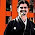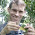## Wednesday, February 6, 2013

### Robinson-Foulds distance and NNI

A colleague just asked me:

Do you know of a way in R to calculate the topological difference between 2 trees as the (ed. minimum) number of nearest-neighbor interchanges required to go from one to the other?

I told him that I'm not sure of the reference for this, but if I remember correctly it is just the Robinson-Foulds distance divided by 2. Indeed, this seems to be the case. Below, I'll demo this. I first simulate a very large tree (I'll explain why in a moment), apply random NNIs using 'phangorn' function rNNI, and then compute RF distance for each tree (using phangorn::RF.dist). Here is the result:

> require(phangorn)
> tree<-rtree(n=10000)
> # one random NNI step
> trees<-rNNI(tree,moves=1,n=20)
> rf<-sapply(trees,RF.dist,tree2=tree)
> rf
 2 2 2 2 2 2 2 2 2 2 2 2 2 2 2 2 2 2 2 2
> # two steps
> trees<-rNNI(tree,moves=2,n=20)
> rf<-sapply(trees,RF.dist,tree2=tree)
> rf
 4 4 4 4 4 4 4 4 4 4 4 4 4 4 4 4 4 4 4 4
> # four steps
> trees<-rNNI(tree,moves=4,n=20)
> rf<-sapply(trees,RF.dist,tree2=tree)
> rf
 8 8 8 8 8 8 8 8 8 8 8 8 8 8 8 8 8 8 8 8
> # 10 steps
> trees<-rNNI(tree,moves=10,n=20)
> rf<-sapply(trees,RF.dist,tree2=tree)
> rf
 20 20 20 20 20 20 20 20 20 20 20 20 20 20 20 20 20 20
 20 20
> # 20 steps > trees<-rNNI(t
ree,moves=20,n=20)
> rf<-sapply(trees,RF.dist,tree2=tree)
> rf
 40 40 40 40 40 40 40 40 40 40 40 40 40 40 40 40 40 40
 40 40

The reason I used a very large starting tree (10,000 tips in this case) was because for small trees, successive random NNIs will cancel each other with some regularity - making the minimum number of NNIs separating two trees (i.e., RF/2) smaller than the number of simulated NNIs. For example:

> tree<-rtree(n=50)
> # four steps
> trees<-rNNI(tree,moves=4,n=20)
> rf<-sapply(trees,RF.dist,tree2=tree)
> rf
 8 8 4 6 8 8 8 8 8 8 8 8 8 8 8 8 8 8 8 8
> # 10 steps
> trees<-rNNI(tree,moves=10,n=20)
> rf<-sapply(trees,RF.dist,tree2=tree)
> rf
 16 20 20 20 18 16 20 20 20 14 20 20 16 16 20 18 20 20
 14 18
> # 20 steps
> trees<-rNNI(tree,moves=20,n=20)
> rf<-sapply(trees,RF.dist,tree2=tree)
> rf
 26 20 22 26 32 28 32 28 34 34 28 24 30 32 34 24 30 38
 26 34

This will eventually even happen for large trees. For fun, here is the some simulation code to compute the relationship between mean RF distance and number of random NNIs for trees of varying size:

# function to simulate rNNIs and return mean RF dist
f1<-function(tree,moves,n=20)
mean(sapply(rNNI(tree,moves,n),RF.dist,tree2=tree))
# function to get the mean RF distance over random trees
f2<-function(moves,ntaxa,nrep=20,nmoves=20)
mean(sapply(rmtree(N=nrep,n=ntaxa),f1,moves=moves, n=nmoves))
# now compute mean RF distance for various NNIs
# across trees of different size
moves<-c(2*1:5,15,20,30,40)
ntaxa<-c(10,20,40,80,160,320)
XX<-matrix(NA,length(moves),length(ntaxa), dimnames=list(moves,ntaxa))
for(i in 1:length(ntaxa))
XX[,i]<-sapply(moves,f2,ntaxa=ntaxa[i])
# plot
plot(c(0,max(moves)),c(0,2*max(moves)),xlab="NNI moves", ylab="mean RF distance",type="l",lty=2, xlim=c(0,max(moves)+0.1*max(moves)),ylim=c(0,2*max(moves)))
for(i in 1:ncol(XX)){
lines(moves,XX[,i],type="b")
text(x=moves[length(moves)],y=XX[length(moves),i], paste("N=",ntaxa[i],sep=""),pos=4,offset=0.3)
}

If any readers know of the reference or proof for this - please post to the comments. (I only did a very casual search for this - it may be an easy reference to find, in which case I apologize.)

#### 4 comments:

1.In fact the NNI and the RF distances are not the same, despite I've seen them being used as if they were equivalent. The reference is this one:

http://citeseer.ist.psu.edu/viewdoc/summary?doi=10.1.1.35.5588

There are also these two more recent ones, that comment on this inequality:

http://www.springerlink.com/content/w811uuqrm7w5hg47/

http://sysbio.oxfordjournals.org/content/61/2/228

One explanation is that when you make tree T2 to be equal to T1 by applying NNIs, some of those NNIs will not change the RF distance (the number of unique edges). Another explanation is that one is hard, and the other is not :)

Your justification for using large trees is correct, but it also prevents us from seing the cases where the distances (legitimately) disagree...

1.How interesting. Thanks for the references.

Basically what you're saying is in the path of NNIs from T2 to T1 that is the minimum length path, some steps:
T2->Ti->Tj->...->T1
don't result in a change in the RF distance to T1? That is Ti & Tj, one NNI apart, might differ from each other in RF but are equidistant from T1?

It would be nice to have an example of this kind of path for the readers of this blog.

2.All steps Ti->Tj have an RF distance of 1, but by a rationale similar to the imperfection of the simulations, they might "cancel out". The NNI distance -- the whole path T2->Ti->...->T1 -- may be larger than looking only at the affected edges. Even if we consider only the most economical path...

I included an example here:
http://blog.leomartins.org/2013/02/the-difference-between-rf-and-nni.html

(A generalization might be a long caterpillar tree where several leaves walk from one end to the other: the NNI distance considers all the 1-step movements of each leaf, while the RF computes the "backbone" path only once.)

I hope it helps...

3.Thanks for sharing this. See my addendum to this thread here.

Note: due to the very large amount of spam, all comments are now automatically submitted for moderation.